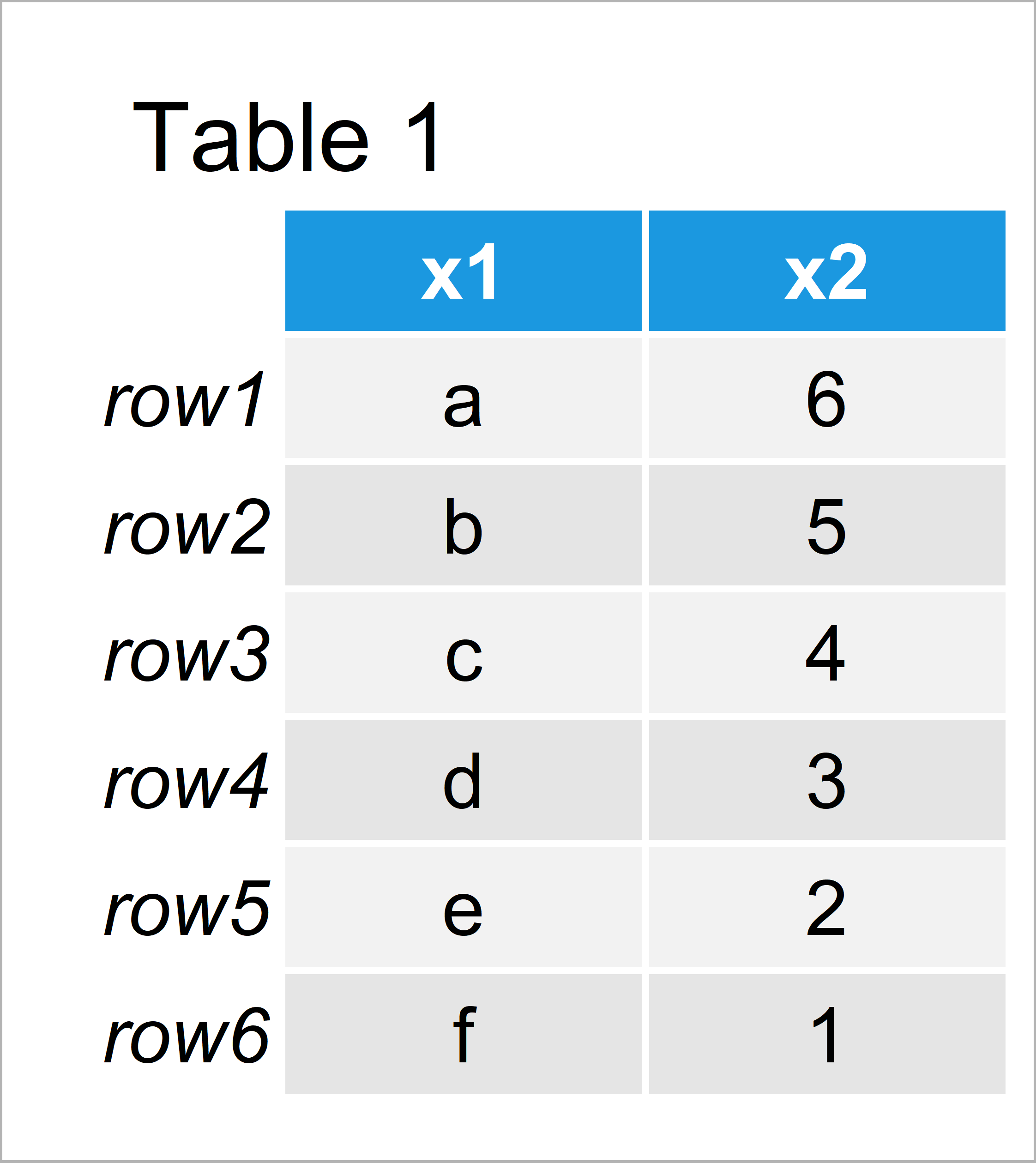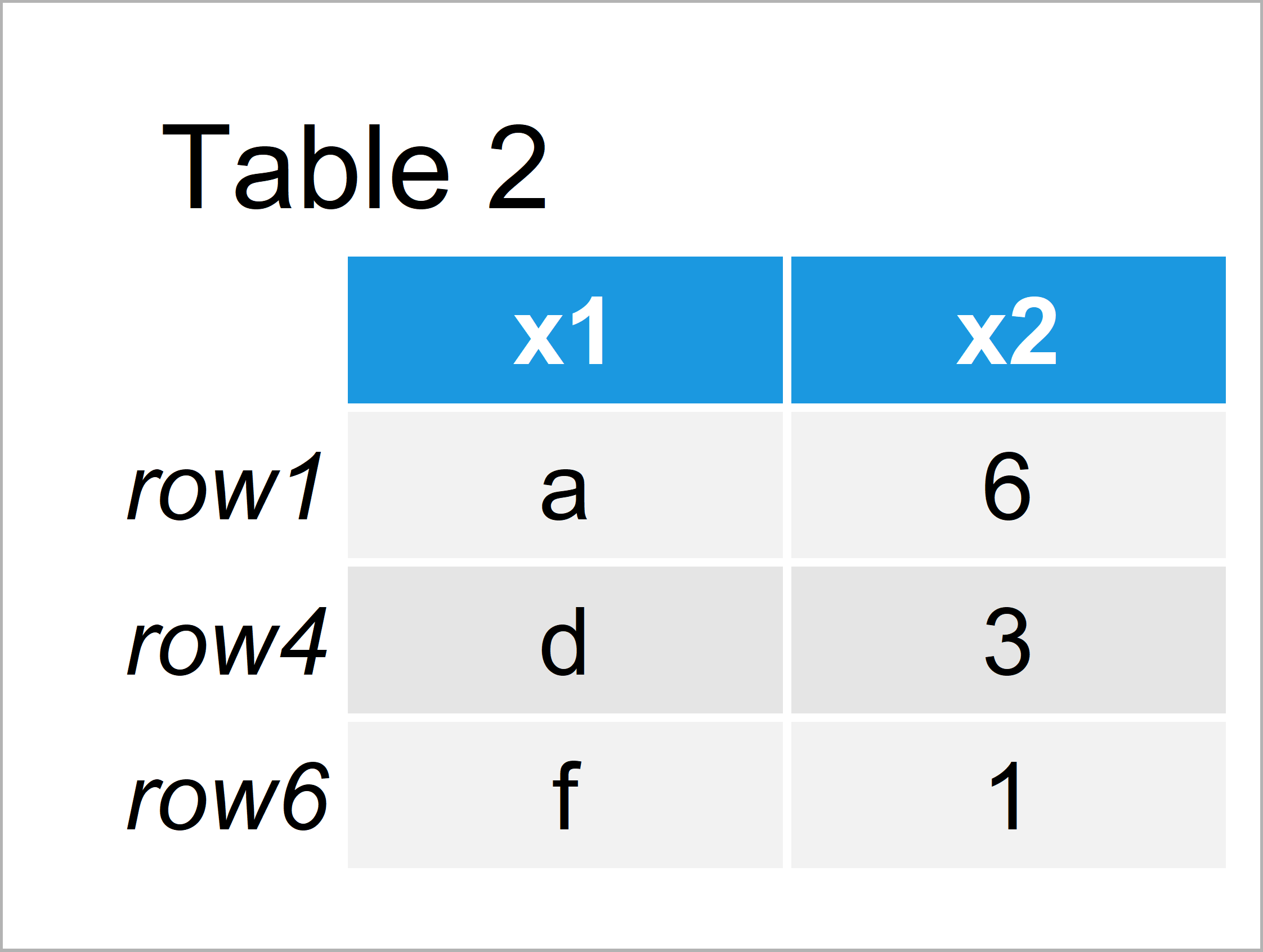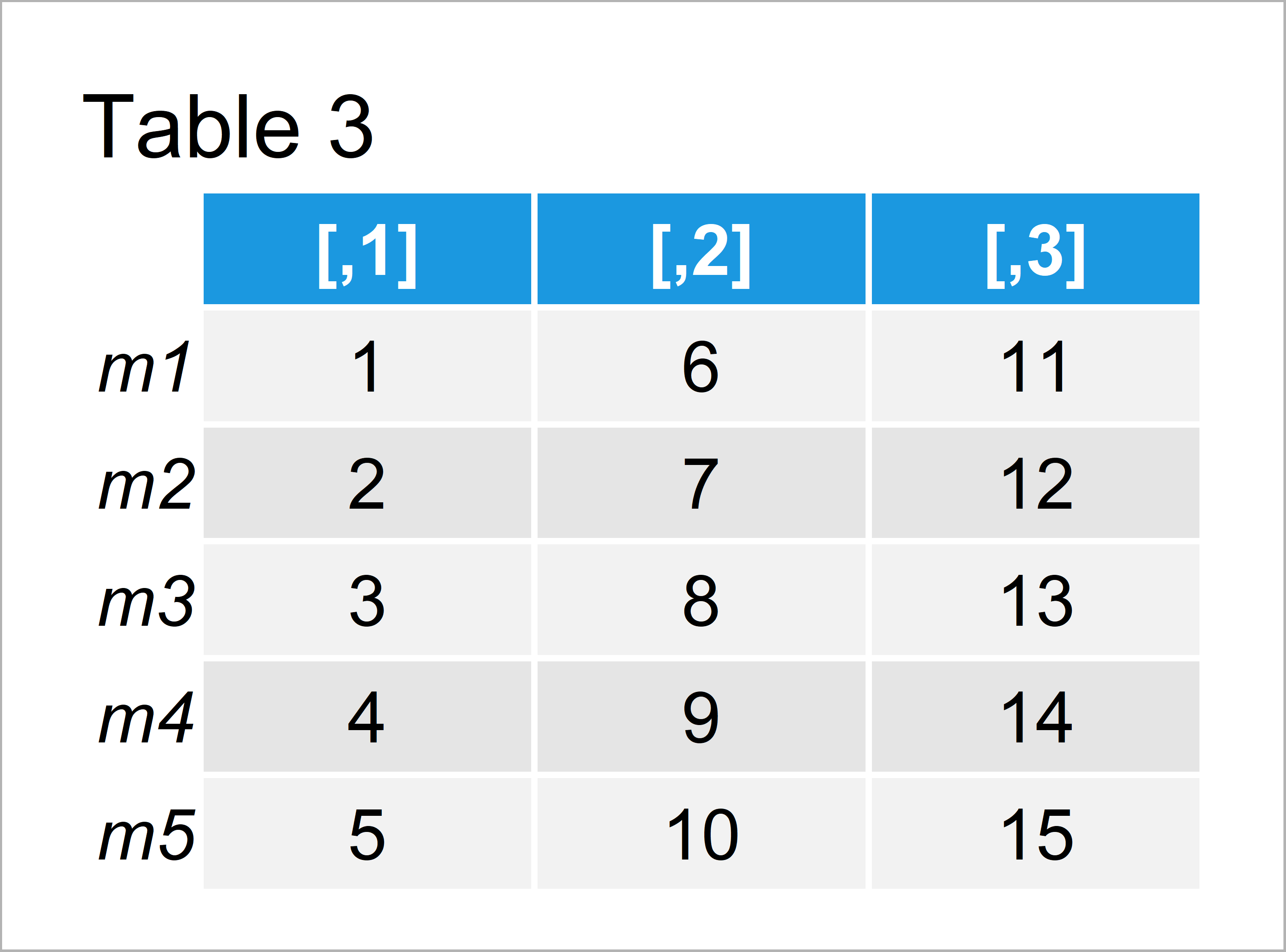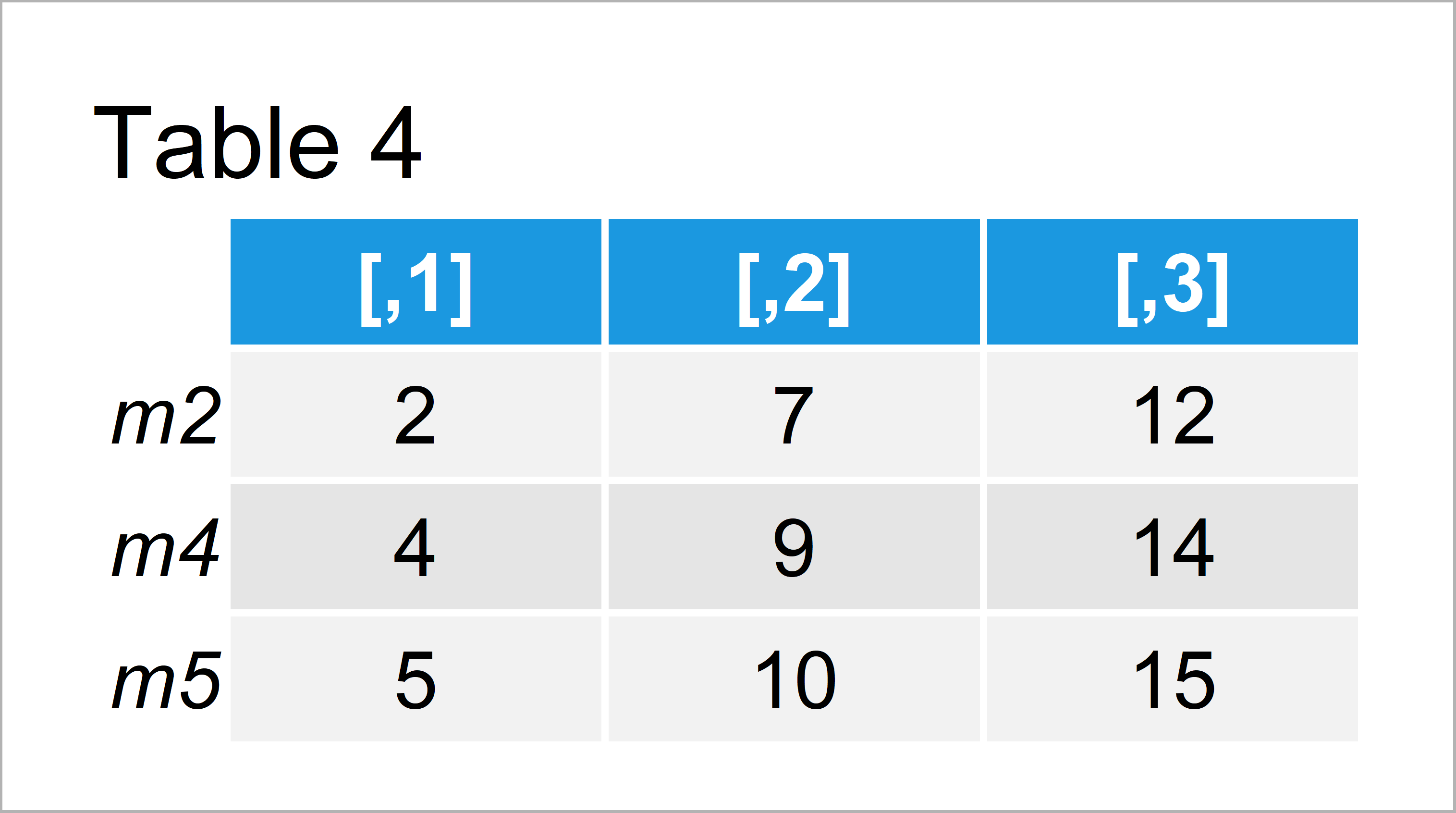# Subset Data Frame and Matrix by Row Names in R (2 Examples)

In this article, I’ll show how to select certain observations based on row names in the R programming language.

The article contains the following content blocks:

Let’s jump right to the programming part.

## Example 1: Extract Certain Data Frame Rows Based On Row Names

In this example, I’ll show how to select particular lines of a data frame based on the row names of this data frame.

First, let’s create an exemplifying data frame in R:

```data <- data.frame(x1 = letters[1:6],                      # Create example data frame
x2 = 6:1)
rownames(data) <- paste0("row", 1:6)                       # Change row names of data frame
data                                                       # Print example data frame```Table 1 illustrates the structure of our data frame. It has six rows and two columns. The rows are named row1-row6.

Next, we have to specify the rows that we want to keep in our data frame subset:

`data_keep_rows <- c("row1", "row4", "row6")                # Specify rows to keep`

The vector data_keep_rows defines the row names of the rows we want to retain.

Now, we can use the %in% operator to eliminate certain rows of our data table:

```data_subset <- data[rownames(data) %in% data_keep_rows, ]  # Extract rows from data
data_subset                                                # Print data frame subset```Table 2 shows the data frame subset we have created with the previous R code. As you can see, we have kept only three rows.

## Example 2: Extract Certain Matrix Rows Based On Row Names

In Example 2, I’ll illustrate how to subset the rows of a matrix based on the row names of this matrix.

Again, we have to create some example data first:

```mat <- matrix(1:15, nrow = 5)                              # Create example matrix
rownames(mat) <- paste0("m", 1:5)                          # Change row names of example matrix
mat                                                        # Print example matrix```Table 3 illustrates how our matrix looks like in the RStudio console.

Now, we have to specify which rows we want to subset:

`mat_keep_rows <- c("m2", "m4", "m5")                       # Specify rows to keep`

Finally, we can use the %in% operator (as we already did in Example 1) to select specific matrix rows based on their row names:

```mat_subset <- mat[rownames(mat) %in% mat_keep_rows, ]      # Extract rows from matrix
mat_subset                                                 # Print matrix subset```The final output is shown in Table 4.

## Video, Further Resources & Summary

Have a look at the following video of the Statistics Globe YouTube channel. I’m explaining the R programming syntax of the present tutorial in the video:

Furthermore, you could read the related R programming articles on https://statisticsglobe.com/.